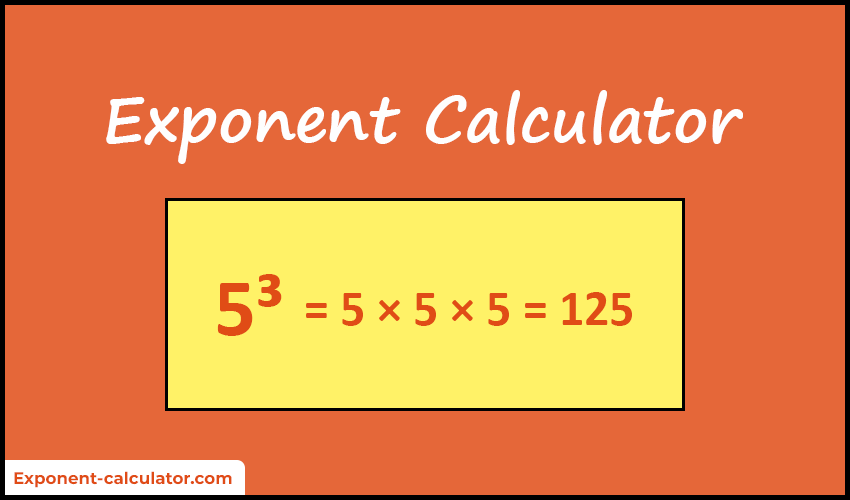# Exponent Calculator

The exponent calculator is designed to determine the exponential expression of any integers and real numbers.

xn = ?

 Base (x) Exponent (n)

Use "e" for scientific notation ( E.g: 4e2, 2e-6, 2.46e15 )